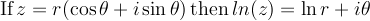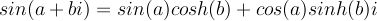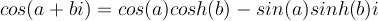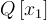# Math2.org Math Tables: Complexity(Math)

## Basic Operations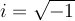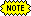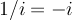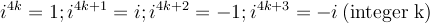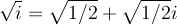## Complex Definitions of Functions and Operations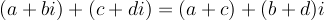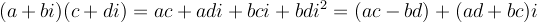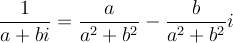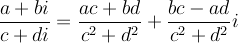(sum of squares)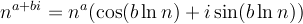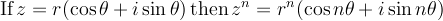(DeMoivre's Theorem)
if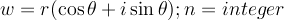then there are n complex nth roots (z) of w for k=0,1,..n-1
$z(k) = r1/n [ \cos \frac{\theta + 2 \pi k}{n} + i \sin \frac{\theta + 2 \pi k}{n} ]$